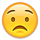# AJAX Where conditions

Hi,

I have a problem with an AJAX backend. I want to filter the results with where conditions.
But if I use an or_where function, the whole table returns.

``````Editor::inst( \$db, 'Munka2', 'ID' )
->fields(
Field::inst( 'ID' ),
...
)
->where( 'Kezdodatum', '2019-02-01', '>=' )
->where( function ( \$q )
{
\$q
->where( 'Folyamat', 'K', '=' )
->or_where( 'Folyamat', 'L', '=' );
} )
->process( \$_POST )
->json();

``````

In this case the fist date condition doesn't work. If I remove the or_where, the date condition works.

Thank You and best regards:
Endre, Szak

## Replies

edited February 2019

Hi @szakendre ,

It looks like your format is incorrect - take a look at the example here.

I suspect it should be:

``````    ->where( function ( \$q ) {
\$q->where( function ( \$r ) {
\$r->where( 'Folyamat', 'K', '=' )
\$r->or_where( 'Folyamat', 'L', '=' );
} ) ;
} )
``````

Hope that does the trick,

Cheers,

Colin

• Dear Colin,

That really did the trick.I'm noob.Thank You...

This discussion has been closed.